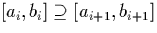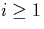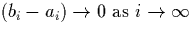Next: Algorithm Design Up: Representations Previous: (Mantissa, Exponent) Representation

# Nested interval Representation

The digit stream representations described in sections 3.3.1 and 3.3.2, and the (mantissa, exponent) representations derived from them, have the property that as we evaluate more digits, the range of possible numbers represented by the stream converges at a constant rate.

When computing the limits of Cauchy sequences as we do in section 5.2, it is useful to use a representation which does not have this property (ie. has a variable rate of convergence). One such representation a stream of nested intervals.

When implementing such a representation, the end points of the intervals may be either rationals or other exact real numbers. The the latter approach has been adopted here for simplicity (because we have already implemented algorithms for exact reals). Each element of the stream x is a nested interval expressed as a pair of numbers as follows:

x = [a1, b1] :: [a2, b2] :: [a3, b3] :: ...

wherefor all values of, and. The semantics of x are defined as

For the purposes of implementing the algorithm to compute the limits of Cauchy sequences, we use the signed binary (mantissa, exponent) representation to hold the end-points of each interval which we can then convert into a single numeral in signed binary (mantissa, exponent) representation as described in section 5.1. An exponent is stored at every end-point of every interval for simplicity, although because of the convergent properties described above, every number in each interval could be represented using the highest exponent seen in the interval at the head of the sequence.Next: Algorithm Design Up: Representations Previous: (Mantissa, Exponent) Representation
Martin Escardo
5/11/2000